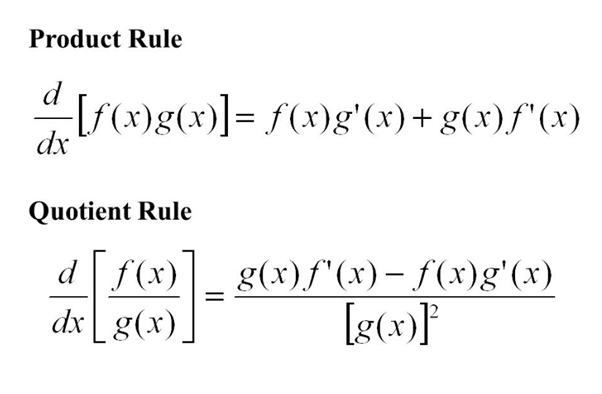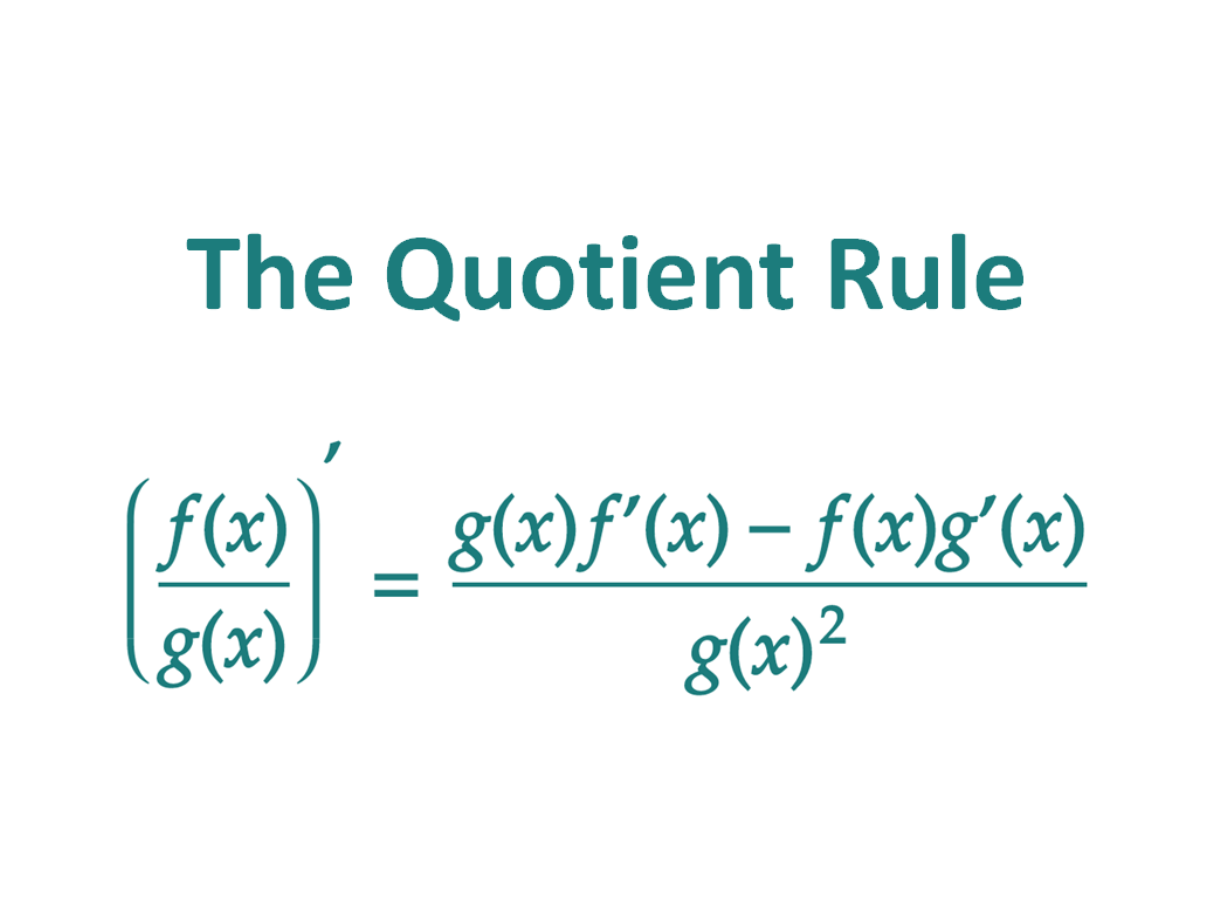# What are quotient and product rules? What are the derivatives and their uses?

Quotient rule is a formulaic application one uses in differentiation problems. So, in this case, one function divides another. There is a particular way in which you must remember the quotient rule. So, begin with the bottom function and end by squaring the same. You can define the quotient rule as the product of the denominator and the derivative of the numerator subtracted from the product of the numerator and the derivative of the denominator. However, all this while the denominator is the square of the original denominator function. It might sound a bit complex but it is not so. We will see how.

The product rule is again a formulaic application one uses in differentiation problems. In this case, the addition of the product of the derivative of the first function with the second function and the product of the derivative of the second function with the first function gives the derivative of the product of the two functions. Hence, one must use the product rule while considering the quotient of the two functions that one has.

## Quotient and product rule derivatives

Quotient rule derivative

So, we use the quotient rule when we need to find the derivative of the quotient of two functions.

Therefore, suppose your function in the numerator if f(x). On the other hand, the function in the denominator is g(x). So, the formula of the quotient rule derivative would be-

d/dx (f(x) / g(x)) = ((g(x) * (d/dx) f(x)) – (f(x) * (d/dx) g(x)) / ((g(x)) ^2)

where d/dx denotes the derivative of the particular term which attaches itself with it. Therefore, d/dx(f(x)) means the derivative of the numerator function. On the other hand, d/dx(g(x)) means the derivative of the denominator function.### Product rule derivative

So, we use the product rule when we need to find the derivative of the product of two functions.

Therefore, suppose one of your functions is f(x). On the other hand, the other function is g(x). So, the formula of the product rule derivative would be-

d/dx (f(x) * g(x)) = (f(x) * d/dx (g(x))) + (g(x) * d/dx (f(x)))

where d/dx denotes the derivative of the particular term which attaches itself with it. Therefore, d/dx (f(x)) means the derivative of the function f(x). On the other hand, d/dx (g(x)) means the derivative of the function g(x).### Chain product and quotient rule

We often confuse ourselves regarding where we should use the chain rule, where the product rule, and where the quotient rule. Moreover, particularly the applications of the chain rule and product rule might be very confusing because they look very similar. So, we use a chain rule when we have to differentiate the function of a function. Therefore, this comes in the format of d/dx ((f(g(x))). So, here x is a function of g(x) while g(x) is a function of f(g(x)). Hence there is a chain of functions and they are not multiplied with each other.

###### On the other hand, we use the product rule when we simply multiply two functions and then need to find out their derivative. Hence, this would be in the format of d/dx ((f(x)) * (g(x))).

However, it is important to note that with an increase in difficulty level in problems, we might need to use chain rule with product or quotient rule. For instance, suppose you have to find the quotient of two functions where the numerator has a chain of functions. So, in this case, you have to apply the quotient rule at first. However, when you have to find out the derivative of the numerator function inside the quotient rule, you have to use the chain rule as well. So, this is how these three works and interlink themselves.### Quotient and product rule examples

Product rule example

Quo. f(t) = (t – 4) (2t^2)

Ans. So, following the product rule,

d/df(t) = d/dt (t – 4) * 2 (t^2) + d/dt (2 (t^2) * (t – 4)

Now, d/dt (t- 4) gives 1 as 4 is constant which yields a 0 on differentiation. On the other hand, d/dt (2(t^2) gives 4t as per the power rules of differentiation.

Therefore, d/df(t) = 2 (t^2) * 1 + 4t (t – 4)

= 2t^2 + 4t^2 – 16t

= 6t^2 – 16t.

= 2t (3t – 8).

Quotient rule example

Quo. f(t) = 4t^2 / (2t – 1)

Ans. So, following the quotient rule,

d/df(t) = ((2t -1) * (d/dt) 4t^2) – (4t^2 * (d/dt) (2t -1)/ ((2t -1) ^2)

Now,

d/dt of 4t^2 yields 8t as per power rules of differentiation. On the other hand, d/dt of 2t- 1 yields 2 because 1 is a constant which yields a zero on differentiation,

Therefore, d/df(t)= ((8t (2t – 1) – (4t^2 * 2))/ (2t – 1) ^2

= ((16t^2 – 8t) – (8t^2)) / (2t – 1) ^2

= (8t^2 – 8t)/ (2t – 1) ^2

= 8t (t – 1)/ (2t – 1) ^2

Quotient and product rule combined

So, now we need to note that problems in calculus are hardly simple. Think in a different light. We use calculus not to just solve sums. We use them for making intricate calculations in Physics or Chemistry where we have to deal with very fine quantities. So, in such cases calculations can hardly be so linear that we can simply apply one formula or rule and get the final result.

Therefore, as the difficulty level of differentiation problems increases, their complexities also equally increase. So, as a result, in most of the cases, you might need to use the quotient and product rule both while solving a single derivative. It is also normal to use chain rule in the process because the functions are hard with one dependent variable.

So, for example in a function like, let us say arbitrarily-

f(t) = 2t (4 (t -1) ^2 – 4t) / t (t -1),

one needs to use the chain rule, quotient rule as well as product rule to find out the derivative properly.

Quotient and product rule practice

So, you can understand no mathematical problem properly if you do not go through enough practice problems. Thereby, enlisting a few here-

### Simpler problems:

1. y= (1 + √x^3) (x – 3 − 23√x) y= (1 + x3) (x – 3 − 2×3)

Ans: So, here we need to convert the roots to fractional exponents.

y= (1+ x^ (3/2)) (x^ (−3) − 2x^ (1/3))

The derivative is then,

dy/dx = ((3/2) x^ (1/2)) (x^ (−3) − 2x^ (1/3)) +(1+x^ (3/2)) (−3x^ (−4) – (2/3) x^ (−2/3)) = −3x^ (−4) – (3/2) x^ (−5/2) – (2/3) x^ (−2/3) – (11/3) x^ (5/6)

1. h(z)= (1 + 2z + 3z^2) (5z + 8z^2 − z^3)

Ans: So, here again we are going to use the product rule to make the derivative-

h′(z) = (2 + 6z) (5z + 8z^2 −z^3) + (1 + 2z + 3z^2) (5 + 16z − 3z^2) = 5 + 36z + 90z^2 + 88z3 − 15z^4

1. g(x) = 6x^2 / (2-x)

Ans: So, using the quotient rule-

g′(x) = 12x (2 − x) − 6×2 (−1) (2 − x) 2 = 24x – 12 (2 − x) ^2

1. R(w) = (3w + w^4) / (2w^2+ 1)

Ans. So, here again we need to use the quotient rule-

R′(w) = (3 + 4w^3) (2w^2 + 1) − (3w + w^4) (4w) / (2w^2 + 1) ^2

= 4w^5 + 4w^3 − 6w^2 + 3 / (2w^2 + 1) ^2

### More difficult problems

1. If f (2) = −8, f′ (2) = 3,g(2) = 17, and g′ (2) = −4, then determine the value of (fg)′ (2)

Ans. So, we already know that the product rule is,

(f * g) ′ (x) = f′(x) * g(x) + f(x) * g′(x)

Now, in this case what we want to know is the value of this when x= 2. Therefore, all that we

need to do is to put this value into the derivative. So, by that, we get-

(fg)′ (2) = f′ (2) * g (2) + f (2) * g′ (2)

So, now that we have already got the formation, we can just put in the values- as simple as that. Therefore,

(fg) ′ (2) = (3) * (17) + (−8) (−4) = 83

1. If f(x) = x^3 * g(x), g (−7) = 2, g′ (−7) = −9, thendetermine the value of f′(−7)

Ans. So here, we don’t know what g(x) is. However, we do have a product of two functions

and hence we can use the product rule to determine the derivative of f(x). Therefore-

f′ (x) = 3x^2 * g(x) + x^3 g′(x)

Now, all that we need to do is put x=−7 into the equation and use the given information to

determine the value of f′ (−7).

Therefore-

f′ (−7) = 3 (−7) ^2 * g (−7) + (−7) ^3 * g′ (−7) = 3 (49) * (2) + (−343) (−9) = 3381